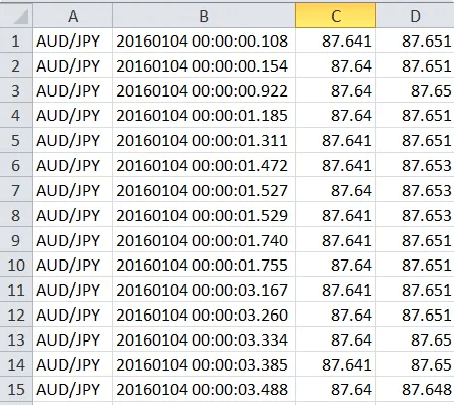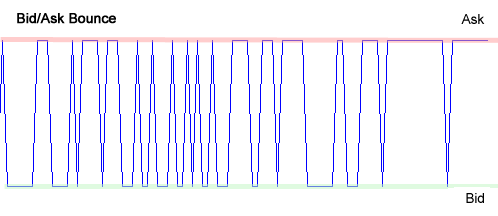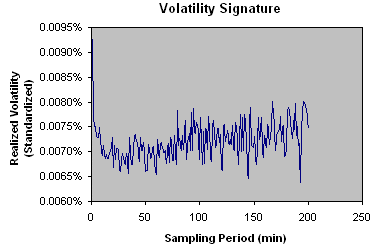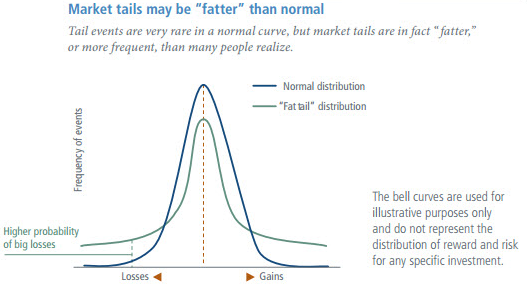# A Glimpse Into Features of High Frequency DataAs the race to zero latency continues, high frequency data, a key component in HFT remains under the scanner of researchers and quants across markets. Beginners to algorithmic trading often find the words high frequency trading (HFT), latency, market microstructure, noise etc. being tossed around on numerous algorithmic trading sites, in research papers, and quant literature. This post aims to unravel some of these terms for our readers. In this post, we will take a brief overview of the features of high frequency data, some of which include:

• Irregular time intervals between observations
• Market microstructure noise
• Non-normal asset return distributions (e.g. fat tail distributions)
• Volatility clustering and long memory in absolute values of returns
• High computations loads and related “Big data” problems

### 1) Irregular time intervals between observations

On any given trading day, liquid markets generate thousands of ticks which form the high frequency data. By nature, this data is irregularly spaced in time and is humongous compared to the regularly spaced end-of-the-day (EOD) data.Example of tick-by-tick data for AUD/JPY pair, Source: Pepperstone.com

High frequency trading (HFT) involves analyzing this data for formulating trading strategies which are implemented with very low latencies. As such it becomes very essential for mathematical tools and models to incorporate the features of high frequency data such as irregular time series and some others that we will outline below to arrive at the right trading decisions. Let us cover some of the other features that define high frequency data.

### 2) Market Microstructure Noise

Market Microstructure Noise is a phenomenon observed with high frequency data that relates to observed deviation of the price from the base price. The presence of Noise makes high frequency estimates of some parameters like realized volatility very unstable. Noise in high frequency data can result from various factors including:
2. Asymmetry of information
3. Discreteness of price changes
4. Order arrival latency

Let us look at the concept of Bid-Ask Bounce, which is one of the causes of Noise.

Bid-Ask bounce - Bid-Ask bounce occurs when the price for a stock keeps changing from the bid price to ask price (or vice versa). The stock price movement takes place only inside the bid-ask spread, which gives rise to the bounce effect. This occurrence of bid-ask bounce gives rise to high volatility readings even if the price stays within the bid-ask window.### 3) Fat tail distributions

High frequency data exhibit fat tail distributions. To understand fat tails we need to first understand a normal distribution. A normal distribution assumes that all values in a sample will be distributed equally above and below the mean. Thus, about 99.7% of all values falls within three standard deviations of the mean and therefore there is only a 0.3% chance of an extreme event occurring.

Many financial models such as Modern Portfolio TheoryEfficient Markets, and the Black-Scholes option pricing model assume normality. However, real market events in the past have shown us that the unpredictable human behavior makes marketplace less than perfect. This gives rise to extreme events and consequently to the fat tail distribution and the consequent risks.

By definition, a fat tail is a probability distribution which predicts movements of three or more standard deviations more frequently than a normal distribution. Quant analysts doing HFT need to model the tail risks to avoid big losses, and hence tail risk hedging assumes importance in HFT.

The plot shown below illustrates a fat tail distribution vis-à-vis normal a distribution.Source: lexicon.ft.com

### 4) Volatility clustering and long memory in absolute values of returns

High frequency data exhibits volatility clustering and long memory effects in absolute values of returns.

Volatility Clustering - In finance, volatility clustering refers to the observation, as noted as Mandelbrot (1963), that "large changes tend to be followed by large changes, of either sign and small changes tend to be followed by small changes."

Long-range dependence (Long memory) - Long-range dependence (LRD), also called long memory or long-range persistence, is a phenomenon that may arise in the analysis of spatial or time series data. It relates to the rate of decay of statistical dependence of two points with increasing time interval or spatial distance between the points. A phenomenon is usually considered to have long-range dependence if the dependence decays more slowly than an exponential decay, typically a power-like decay.

### 5) High computations loads and related “Big data” problems

HFT players rely on microsecond/nanosecond latency and have to deal with enormous data. Utilizing big data for HFT comes with its own set of problems. HFT firms need to have the latest state-of-the-art hardware and latest software technology to deal with big data, which otherwise can increase the processing time beyond the acceptable standards.

### To Conclude

These were some of the features underlying high-frequency data that HFT models need to take into account. To learn more on the subject, you can watch the webinar, “Alpha Generation: Controlling Intraday Risk Profile” hosted by QuantInsti® and conducted by Stephanie Toper, Director of Portfolio Analytics, PortfolioEffect. The webinar was held on January 10, 2017.

If you want to learn various aspects of Algorithmic trading then check out the Executive Programme in Algorithmic Trading (EPAT®). The course covers training modules like Algorithmic & Quantitative Trading, Statistics & Econometrics, and Financial Computing & Technology. Enroll now!